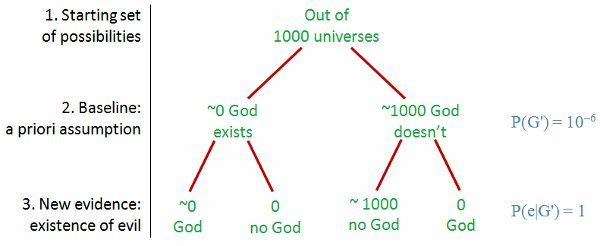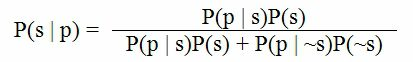# Using Bayes Theorem to Decide How Likely the Jesus Miracle Stories

Using Bayes Theorem to Decide How Likely the Jesus Miracle Stories October 23, 2015

Christianity makes some fanciful claims: Mary was a virgin when she gave birth to Jesus. Jesus turned water into wine. He raised Lazarus from the dead and was resurrected from the dead himself. He is God, one with the creator of the universe.

One response to these claims is simply to dismiss them. We could put them in the same bin as the tall tales from other mythologies. Let’s instead see how probability can be applied to questions like this. Christians and non-Christians won’t easily agree because they won’t agree on the individual probabilities. Still, an understanding of conditional probability will give us a powerful analytical tool to at least better understand these claims.

Richard Carrier (whose Skepticon video was helpful in my understanding of this material) says that conditional probability “is the mathematical model for all correct reasoning about empirical claims.”An example: medical test

Let’s imagine a test for a disease that is 95% accurate. That is, it is positive 95% of the time for someone who has the disease and negative 95% of the time for someone who doesn’t. Now imagine a common disease—10% of the population has it, so in a thousand people, 100 have it and 900 don’t.

Now give those thousand people the test. For the 100 sick people, the test gives 95 positives and 5 (false) negatives. And for the 900 healthy people, it gives 855 (900 × 0.95) negatives and 45 (false) positives.

Suppose the test says that you have the disease. How worrisome is that?

You must be in either of the two groups of people with positive test results. You’re either one of the 95 who actually do have it or one of the 45 who don’t but got a false positive. The chance that you’re sick is the number of sick people who test positive divided by the total number of positives: 95/(95 + 45) = 0.68.

The probability is 68% that you have the disease.

Let’s recap: what’s the probability that you (or any random person) has the disease? 10%. But what’s the probability given that you have a positive test result? It’s 68%. That’s conditional probability—the likelihood of something given (conditional upon) something else, some additional information.

Make the test ten times more accurate and a positive test results means a 96% chance that you have the disease. Instead—and here’s where it gets interesting—make the disease one tenth as common and your likelihood of having the disease given a positive test result is 16%. Make it very rare—one in a million—and that likelihood becomes just 0.005%.*

Visual approach to the same problem

Let’s explore the original problem but visually this time.This tree is just a recap of the previous problem: we start with 1000 people, then divide them into two groups based on what we know initially (the probability of a person being sick is 0.1), and finally process this with new information, the test whose probability of a right answer is 0.95.

Applying probability to the God question

Let’s move on to the God question (I’m using an example from Richard Carrier’s video).We start with 1000 universes, places where we imagine God to exist or not. In step 2, our initial assumption about the God claim is to be generous. Knowing nothing about this “God” guy, let’s start by saying that the likelihood of his existence is 50% (P(G) means “probability of God”). In step 2, this gives us two possibilities, with 500 universes in each.

In step 3, we add our new evidence. In the medical example, the new evidence was the result of a test, and here it’s the existence of evil in our world—birth defects, natural disasters that kill thousands, slavery and other immoral institutions, and so on. This evil exists, and yet no god is doing anything about it. What is the likelihood that a benevolent God could exist but still accept the evil in our world?

We have plenty of examples of benevolent beings: the noblest humans. They’re not perfect, but we could assume that a perfectly benevolent being would be at least as benevolent as a good human. Try to imagine a benevolent human (1) who could prevent bad from happening, (2) wouldn’t be harmed for taking this action, but (3) didn’t do anything. That’s pretty inconceivable. Let’s say that the probability of this happening is one in a million. Let’s be conservative and assign the same probability of standing by and doing nothing to a perfectly benevolent god.

That’s the P(e|G) = 10–6 in the diagram above: the probability (P) of the evidence of evil (e) given (|) the existence of God (G) is one in a million (10–6).

The 500 universes on the left side of the tree have to be divided given the probability of such a god existing given the existence of evil. Only one in a million could have a god (~0 means “almost zero”).

It’s easier on the right side of the tree. The likelihood of evil existing in a godless universe is 1.

Conclusion: the existence of evil makes God very improbable.

But … but God could have an excuse

In response, the Christian may say that God has an excuse for not acting. Yes, he’s benevolent, but he’s also omniscient, and our finite minds must simply be unable to understand the justification for his inaction. (This is the Hypothetical God Fallacy—starting with a presupposition of God’s existence—but let’s ignore that for now.)

“God works in mysterious ways” doesn’t help the Christian position, and the tree shows why.Consider step 3. The conditional probability is now 1. The apologist assumes some unspecified, inconceivable (by our finite brains) reason why God has his own justifiable reasons for allowing evil. But this means we’re looking for something else. We’ve gone from searching for God (G) to searching for “God who has unspecified, inconceivable reasons to allow evil” (G′).

As you can see from step 2, this simply moves the problem around. We had nothing to go on before, so we just assigned a generous 0.5 probability for God (P(G) = 0.5). But now we have a more refined goal that can be evaluated. Now, we’re looking for a very particular God (G′), a very unlikely God, a one-in-a-million God.

Conclusion: making excuses for God makes him less likely. First you must imagine (despite the lack of evidence) supernatural beings, then those with sufficient power to create the universe (deities), then assume that there are benevolent ones that interact with us, then imagine this one-in-a-million deity who has this inconceivable excuse to allow evil, even gratuitous evil like agonizing birth defects in animals.

The mathematics of conditional probability has been applied here to the question, How likely is God given the existence of evil? We could also ask, How likely is the virgin birth given the existence of other virgin birth stories that preceded Jesus that would’ve been known in Palestine? Or, How likely is the resurrection given the existence of stories of other dying-and-rising gods?

(I respond to the book The Probability of God here. That discussion looks at the many other reasons why the Christian god claim is unlikely besides the Problem of Evil.)

This approach will probably never resolve a debate between a Christian and a non-Christian because they won’t be able to agree on probabilities. However, it does give structure to the argument and highlights the unknowns.

Oh, I know He works in mysterious ways,
but if I worked that mysteriously I’d get fired.
— caption for Bob Mankoff cartoon

(This is an update of a post that originally appeared 1/14/13.)

Appendix: Bayes’ Theorem

We have been using Bayes’ Theorem, though it is more commonly expressed as an equation. To see that this tree structured approach is an equivalent (though more intuitive) approach to the equation, let’s convert the medical test example above into equation form.

In that example, we first imagine a population of 1000 people and then (step 2) use the likelihood of the disease (10%) to divide that population into sick and well and then (step 3) further divide those populations into those who got positive and negative test results.

Our goal is P(s | p), the probability (P) of being sick (s) given (|) a positive test result (p). Bayes Theorem says that this is computed as follows:where ~s = the probability of not being sick.

This may look imposing, but you’re already familiar with these terms. Look at the numerator first, a measure of how likely s (being sick) is:

• P(p | s) = the probability of a positive result given that you’re sick = 0.95 (that is, a likelihood of 95%)
• P(s) = the probability of a random person being sick = 0.1 (the incidence is 10% in the population)

The denominator measures all possible results, your being sick and your being well. It’s the sum of the numerator (the sick likelihood) and its opposite (the not-sick likelihood), which is composed of:

• P(p | ~s) = the probability of a positive test result given that you’re not sick (that is, a false positive), which is 0.05 (our example was simple, with false positives and false negatives both at 5%, but in the general case they could be different)
• P(~s) = the probability of not being sick = 0.9. This one is not a variable since P(~s) = 1 – P(s).

Put these values into the equation: 0.95×0.1/(0.95×0.1 + 0.05×0.9) = 0.67857. This is what we got above with the simpler and more intuitive 95/(95 + 45).

* Here is the math behind those probabilities:
99.5/(99.5 + 4.5) = 0.957
9.5/(9.5 + 49.5) = 0.161
1/(1 + 20,000) = 0.00005

Photo credit: Wikimedia

Browse Our Archives

error: Content is protected !!